HLIBpro  2.3.1
Algebraic Clustering

In some applications geometrical data is not available or was lost in some previous computation step. Fortunately, there is another source for describing the relation between indices: the system matrix. In case of a sparse matrix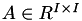over the index set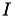, a (matrix) graph can be constructed with nodes corresponding to indices and edges in case of a non-zero matrix entry, e.g.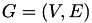with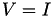and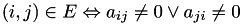Please note, that the symmetrised matrix graph is used, leading to an undirected graph.

By partitioning the graph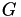, the index setis divided into subset and hence, a cluster tree is constructed by recursivly applying the procedure. This method provides a purely algebraic way for constructing H-matrices.

Before starting with clustering the index set, the sparse matrix has to be available:

TSparseMatrix S;
// ... fill matrix S

# Graph Partitioning

The partitioning of graphs is a standard problem in graph theory. The aim is to split the graph into two or more sub graphs, such that the number of edges connecting the parts is minimized. Albeit this problem is NP-hard, good approximation algorithms have been developed and are available in the form of open source libraries, e.g. METIS or Scotch.

While being able to use these external software libraries, 𝓗𝖫𝖨𝖡𝗉𝗋𝗈 also contains two graph partitioning algorithms:

• BFS partitioning: a variation of the Gibbs-Poole-Stockmeyer algorithm using breadth-first search to flood the graph from two boundary nodes,
• ML partitioning: an implementation of multi-level graph partitioning with random or heavy-edge matching.

In both cases, the Fiduccia-Mattheyses algorithm is applied to optimise the results.

THe actual cluster tree construction algorithms in 𝓗𝖫𝖨𝖡𝗉𝗋𝗈 rely on these graph partitioning strategies, which are implemented in the following classes:

Remarks
The availability of the last two classes depends on the configuration of 𝓗𝖫𝖨𝖡𝗉𝗋𝗈 while compiling the source code.

# Cluster Tree Constructor

The standard cluster tree construction algorithm is implemented in TAlgCTBuilder and uses a graph partitioning strategy to construct a binary cluster tree.

TMLAlgPartStrat part_strat;
TAlgCTBuilder ct_builder( & part_strat, nmin );
auto ct = ct_builder.build( S );

The second argument for the cluster tree constructor defines the size of leaves in the cluster tree, e.g. no leaf with at most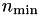indices will be further partitioned. By default,is of size 20 to 40.

In many applications, e.g. if a LU factorisation is planned, a more efficient 𝓗-arithmetic can be obtained by using nested dissection instead of standard bisection for clustering. TNDAlgCTBuilder uses a given cluster tree constructor and extends it by computing an interface (or vertex separator) between the sub graphs, which decouples to indices. The prodedure is applied recursivly on the sub graphs, whereas the nodes in the interface are the divided using the predefined clustering algorithm, e.g. usually bisection.

TMLAlgPartStrat part_strat;
TAlgCTBuilder ct_builder( & part_strat, nmin );
TNDAlgCTBuilder nd_ct_builder( & ct_builder, nmin );
auto ct = nd_ct_builder.build( S );

Another variation from the bisection algorithm uses a predefined partitioning to divide the indices of the root of the cluster tree. This is typically used, if the underlying problem already has a block structure, which shall also be present in the 𝓗-matrices. The user supplied partition is defined by an array of the same size as the number of indices and the value at position i defines the block index i should belong to.

std::vector< idx_t > partition( nindices );
for ( size_t i = 0; i < nindices; ++i )
nindices[i] = ( i % 2 );
TMLAlgPartStrat part_strat;
TAlgCTBuilder ct_builder( & part_strat, nmin );
TPartAlgCTBuilder part_ct_builder( & ct_builder, partition, nmin );
auto ct = part_ct_builder.build( S );

Again, this algorithm extends a given cluster tree constructor, thereby providing a chaining, e.g. using nested dissection for all other levels beside the first:

TMLAlgPartStrat part_strat;
TAlgCTBuilder ct_builder( & part_strat, nmin );
TNDAlgCTBuilder nd_ct_builder( & ct_builder, nmin );
TPartAlgCTBuilder part_ct_builder( & nd_ct_builder, partition, nmin );